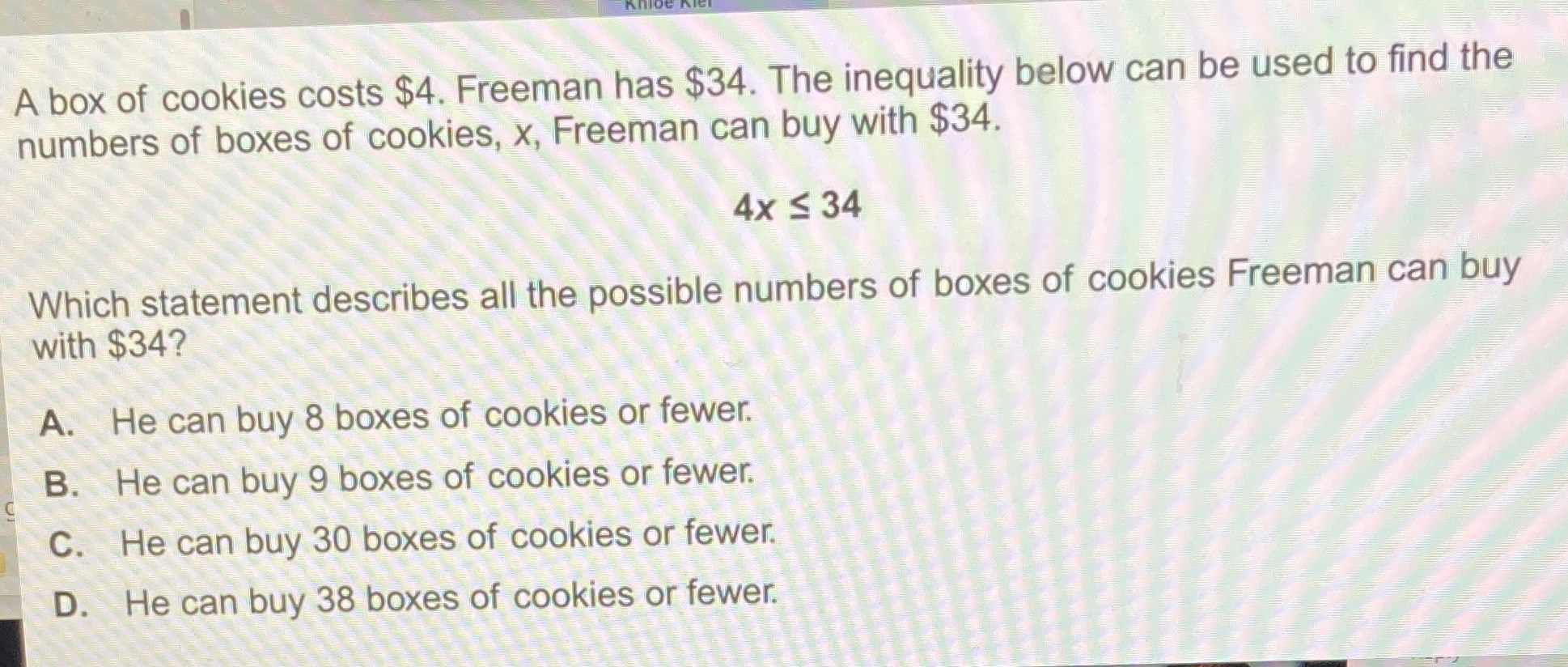### ¿Todavía tienes preguntas de matemáticas?

Pregunte a nuestros tutores expertos
Algebra
PreguntaA box of cookies costs $$\ 4$$ . Freeman has $$\ 34$$ . The inequality below can be used to find the numbers of boxes of cookies, $$x ,$$ Freeman can buy with $$\ 34 .$$

$$4 x \leq 34$$

Which statement describes all the possible numbers of boxes of cookies Freeman can buy with $$\ 34 ?$$

A. He can buy $$8$$ boxes of cookies or fewer. B. He can buy $$9$$ boxes of cookies or fewer. C. He can buy $$30$$ boxes of cookies or fewer. D. He can buy $$38$$ boxes of cookies or fewer.